CATEGORIES:

# Triple Product in Coordinates. Given three vectors , , and , let us express the triple product of these vectors in terms of their coordinates. Consider the triple product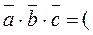.

The vector product equals.

Taking its inner product with, we obtain;

this is a third – order determinant expanded along the last line, i.e.,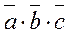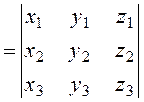.

Thus, the triple product of three vectors equals the third – order determinant of the composed of the coordinates of these vectors.

Example 1. Determine the volume of a pyramid ABCD from the coordinates of its vertices.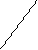D(1;5;2) B(–1;1;3) A(1;2;0) C(0;2;–3) Compose the vectors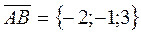,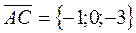,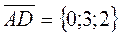.

Let us find the volume of a pyramid by the formulas proved above:The triple product of coplanar vectors equals zero.

The triple product equals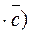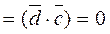, because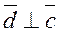.

Thus, the coplanarity condition is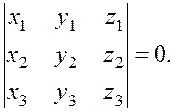Example 2. Show that the four points А(1;2;–1), В(0;1;5), С(–1;2;1), and D(2;1;3) belong to the same plane.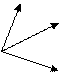B C A D Compose the vectors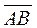={–1;–1;6},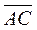={–2;0;2},={1;–1;4}.

To show that they are coplanar, we find the triple product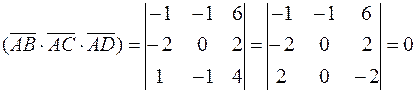.

Thus, the four points belong to the same plane.

Date: 2015-01-02; view: 1517

 <== previous page | next page ==> Consider vectors | Ellipse
doclecture.net - lectures - 2014-2023 year. Copyright infringement or personal data (0.011 sec.)